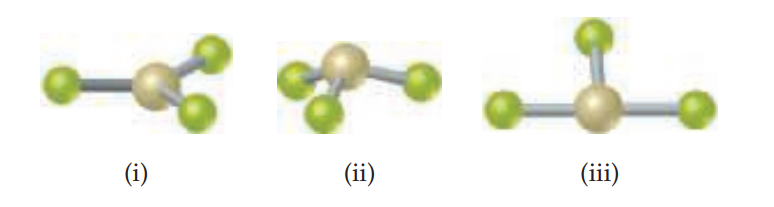×
Get Full Access to Chemistry: The Central Science - 14 Edition - Chapter 9 - Problem 9.27
Get Full Access to Chemistry: The Central Science - 14 Edition - Chapter 9 - Problem 9.27

×

# ?The figure that follows shows ball-and-stick drawings of three possible shapes of an AF3 molecule. (a) For each shape, give the electron-domISBN: 9780134414232 1274

## Solution for problem 9.27 Chapter 9

Chemistry: The Central Science | 14th Edition

• Textbook Solutions
• 2901 Step-by-step solutions solved by professors and subject experts
• Get 24/7 help from StudySoup virtual teaching assistantsChemistry: The Central Science | 14th Edition

4 5 1 411 Reviews
26
2
Problem 9.27

The figure that follows shows ball-and-stick drawings of three possible shapes of an AF3 molecule.

(a) For each shape, give the electron-domain geometry on which the molecular geometry is based.

(b) For each shape, how many nonbonding electron domains are there on atom A?

(c) Which of the following elements will lead to an $$\mathrm{AF}_{3}$$ molecule with the shape in

(ii): Li, B, N, Al, P, Cl?

(d) Name an element A that is expected to lead to the $$\mathrm{AF}_{3}$$ structure shown in (iii).Text Transcription:

AF_3

Step-by-Step Solution:

Step 1 of 5) In a two-dimensional lattice, the unit cells can take only one of the five shapes shown in Figure 12.4. The most general type of lattice is the oblique lattice. In this lattice, the lattice vectors are of different lengths and the angle g between them is of arbitrary size, which makes the unit cell an arbitrarily shaped parallelogram. The square lattice, rectangular lattice, hexagonal lattice,** and rhombic lattice have a unique combination of g angle and relationship between the lengths of lattice vectors a and b (shown in Figure 12.4). For a rhombic lattice an alternative unit cell can be drawn, a rectangle with lattice points on its corners and its center (shown in green in Figure 12.4). Because of this the rhombic lattice is commonly referred to as a centered rectangular lattice. The lattices in Figure 12.4 represent five basic shapes: squares, rectangles, hexagons, rhombuses (diamonds), and arbitrary parallelograms. Other polygons, such as pentagons, cannot cover space without leaving gaps, as Figure 12.5 shows.

Step 2 of 2

## Discover and learn what students are asking

Calculus: Early Transcendental Functions : Slope Fields and Eulers Method
?In Exercises 1-8, verify the solution of the differential equation. Solution D

Statistics: Informed Decisions Using Data : Tests for Independence and the Homogeneity of Proportions
?What’s in a Word? In a recent survey conducted by the Pew Research Center, a random sample of adults 18 years of age or older living in the continenta

Unlock Textbook Solution